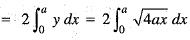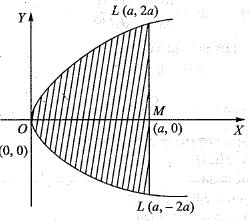Courses

# Integral Calculus -8

## 20 Questions MCQ Test Topic-wise Tests & Solved Examples for IIT JAM Mathematics | Integral Calculus -8

Description
This mock test of Integral Calculus -8 for Mathematics helps you for every Mathematics entrance exam. This contains 20 Multiple Choice Questions for Mathematics Integral Calculus -8 (mcq) to study with solutions a complete question bank. The solved questions answers in this Integral Calculus -8 quiz give you a good mix of easy questions and tough questions. Mathematics students definitely take this Integral Calculus -8 exercise for a better result in the exam. You can find other Integral Calculus -8 extra questions, long questions & short questions for Mathematics on EduRev as well by searching above.
QUESTION: 1

### The area of the cardioid r = a (1 - cos θ) is given by:

Solution:

The given curve is
r= a(l - cos θ)
The required area A is given by
A = 2 x area ABOA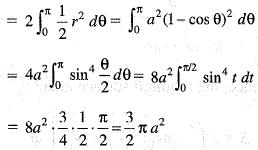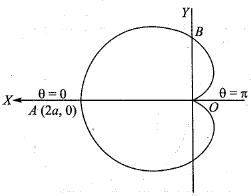QUESTION: 2

### The volume of the solid generated by the revolution of r = 2a cos θ about the initial line is given by:

Solution:

The equation of the given curve is
r= 2a cos θ,
which is a circle with centre (a, 0) and radius a.
Hence The required volume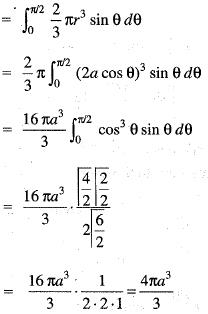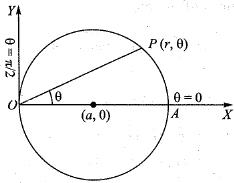QUESTION: 3

### The surface area of the solid generated by the revolution of the curve x = a cos3t, y = a sin3 t about x-axis is given by:

Solution:

The equation of the given curve is
x = a cos3 t,y = a sin3 t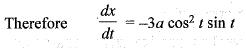Hence, the required surface area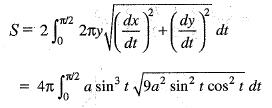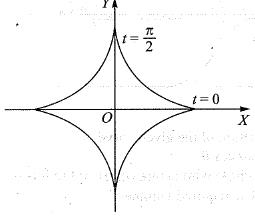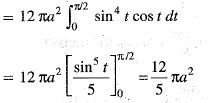QUESTION: 4

The length of the arc of the curve r = a eθ cotα  between the point whose radii vectors are given by r1 and r2 is:

Solution: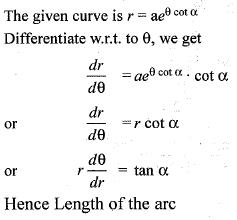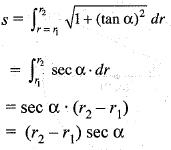QUESTION: 5

The area of the ellipse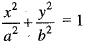is given by:

Solution: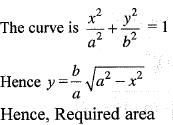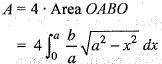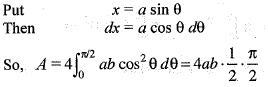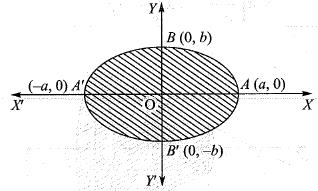QUESTION: 6

The segment o f the circle x2 + y2 = a2 cut off by the chord x = b (0 < b < a) revolves about the x-axis and generates the solid known as a segment of a sphere. The volume of this solid is:

Solution:

The curve bounded by x2 + y2 = a2 and chord x = b revolved about x-axis, then
The required volume is given by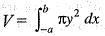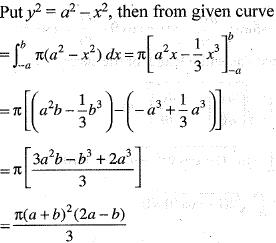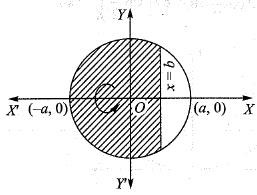QUESTION: 7

The surface area of the solid generated by the revolution of the curve r2 = a2 cos 2θ about a tangent at the pole is given by:

Solution:

Let P(r, θ) be a point on the lemniscate
r2 = a2 cos 2θ
Let OT be a taneent at the pole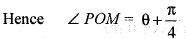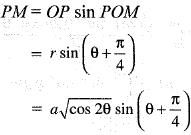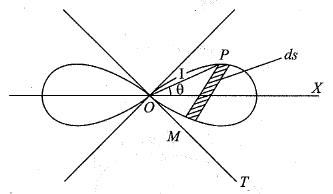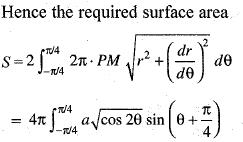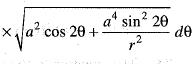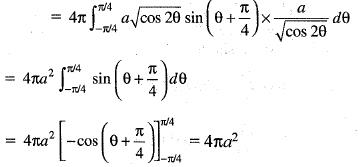QUESTION: 8

The area bounded by the curve y = sin x, x-axis and the lines x = 0, x = π is revolved about y-axis. The surface of revolution is equal to:

Solution:

The equation of the curve is y = sin x.
Hence The required surface area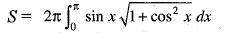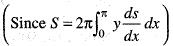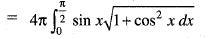Put cos x = t then sin x dx - dt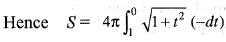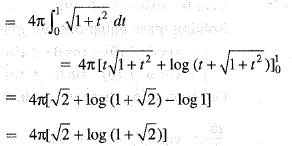QUESTION: 9

The surface of the solid formed by the revolution o f the cardioid r = a (1 + cos θ) about the initial line is given by:

Solution:

The equation of the cardioid is
r = a (1 + cos θ)
The curve is symmetrical about the initial line. The required surface area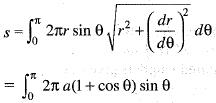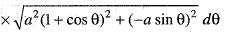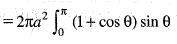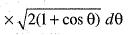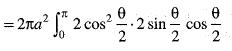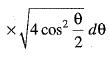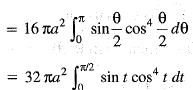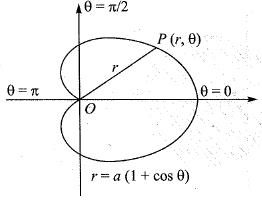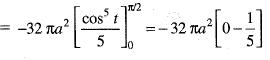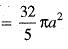QUESTION: 10

The length of the are of the curve
x sin θ + y cos θ = f' (θ),
x cos θ - y cos θ = f " (θ)
is given by:

Solution:

The equations of the given curve are
x sin θ + y cos θ = f' (θ),
x cos θ - y cos θ = f " (θ)
Solving these equations, we get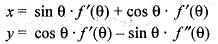Differentiating these w.r. to θ, we get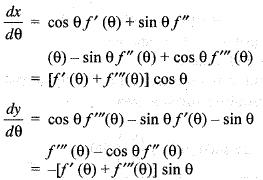Hence the required length is given by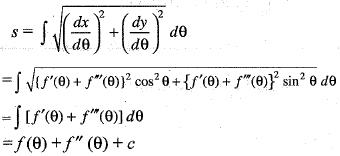QUESTION: 11

The volume of the solid generated by the revolution of the cardioid r = a(1 + cos θ) about the initial line is given by

Solution:

The curve is r = a (1 + cos θ)
Required volume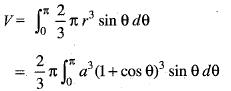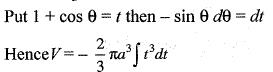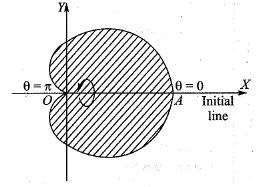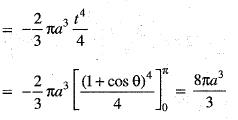QUESTION: 12

The ratio of areas bounded by the curve a4y2 = x5(2a -x) and the circle of radius a is:

Solution:

The area of curve =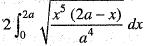Put x = 2 a sin2 θ
Then dx = 4a sinθ cosθ dθ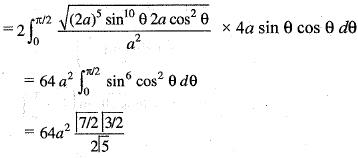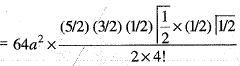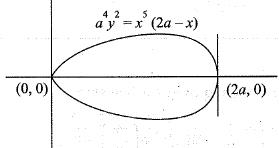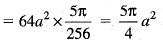Hence, required ratio =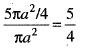QUESTION: 13

By taking (a, 0) as the fixed point, the intrinsic equation of the astroid x2/3 + y2/3 = a2/3 is given by:

Solution:

The equation of the given curve is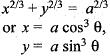Then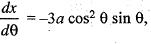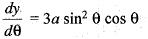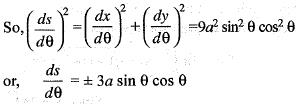Since, (a, 0) is taken as a fixed point, therefore s increases as θ increases.
Hence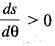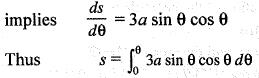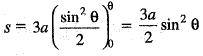..(i)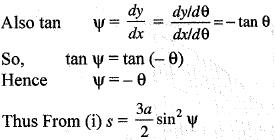QUESTION: 14

The area enclosed by the curve r2 = a2 sinθ is given by:

Solution:

The equation of the given curve is
r2 = a2sinθ
Required area
= Area of OBACO
= 2 x Area OBAO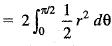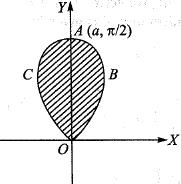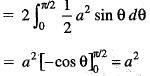QUESTION: 15

The volume of the solid generated by the revolution of the lemniscate r2 = a2 cos2θ about the line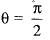is given by:

Solution:

The required volume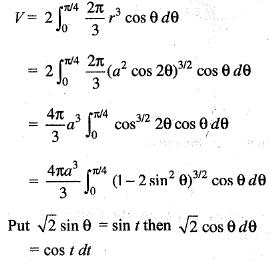Thus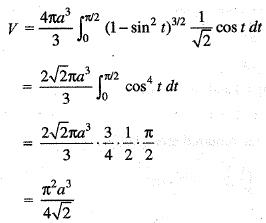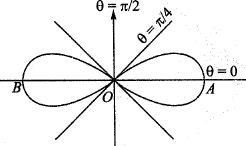QUESTION: 16

If f(x) is continuous in [3, 7], then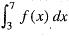is equal to:

Solution:

We have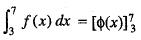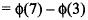QUESTION: 17

The area bounded by the curves y2 = 9x, x - y + 2 = 0 is given by:

Solution:

The equations of the given curves are
y= 9x ...(I)
x - y + 2 = 0 ...(II)
The curves (i) and (ii) intersect at A(1, 3) and B(4, 6) Hence The required area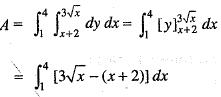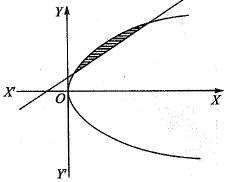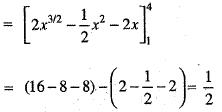QUESTION: 18

The area bounded by the curve y2 = x3 and the line y = 2x is given by:

Solution:

The given curves are
y2 = x3 ...(i)
y = 2x ...(ii)
Solving (i) and (ii), we get
x = 0, x = 4
Hence the required area OPAQO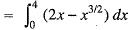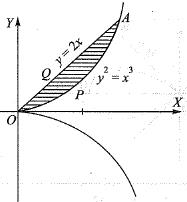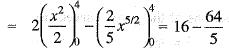= 16/5

QUESTION: 19

The area bounded by the curve x2 = y and y2 = x is given by:

Solution:

Given equations of the curves are
y2 = x ...(i)
x2 = y ...(ii)
Solving these equations, we get
x = 0, x = 1
Hence required area OPAQO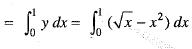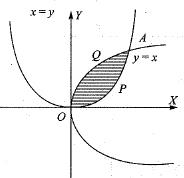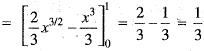QUESTION: 20

The area bounded by the parabola y2 = 4ax and its latus rectum is given by

Solution:

The equation of the parabola is
y2 = 4 ax
We have to find the area OL'MLO
Hence Area OL'MLO = 2 • Area OMLO